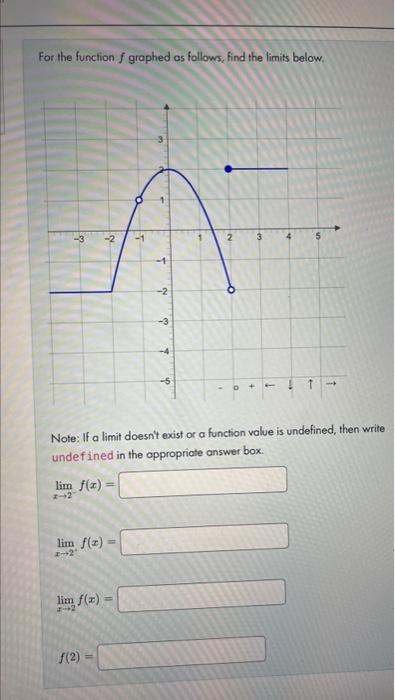Home / Expert Answers / Calculus / for-the-function-f-graphed-as-follows-find-the-limits-below-note-if-a-limit-doesn-39-t-exist-pa887

# (Solved): For the function $$f$$ graphed as follows, find the limits below. Note: If a limit doesn't exist ...For the function $$f$$ graphed as follows, find the limits below. Note: If a limit doesn't exist or a function value is undefined, then write undef ined in the appropriate answer box. $\lim _{x \rightarrow 2^{-}} f(x)=$ $\lim _{x \rightarrow 2^{+}} f(x)=$ $\lim _{x \rightarrow 2} f(x)=$

We have an Answer from Expert